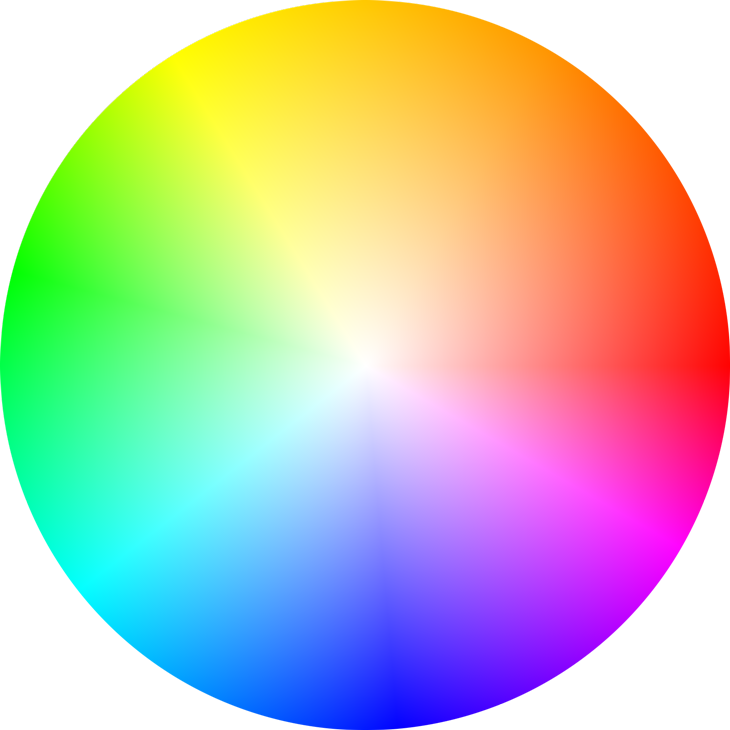## Recommended Posts

I need a way to allow the user to select a colour by clicking on a certain point of a wheel.

The colour wheel that I am using looks like this (Sorry for huge image) :I would like a way for the user to be able to click somewhere on the wheel, and for phaser to be able to get the hex value of that point.  I know that this can be done with canvas but my game uses webGL as well so I need the solution to work with both of possible.

Thanks for any help##### Share on other sites

Well first you need to render the color wheel. A simple sprite will do, but you can also do it via shader, with no actual graphics used anywhere.

You need to register click on the general area of color wheel, it's precise location. First you check the distance to color wheel center through common euclidean distance equation, that would be saturation value. You immediately divide it by color wheel radius, to normalize it. If it's greater than 1, then click was outside of color wheel. But you may want to cap it to 1 so that stray clicks count anyway.

Second, you measure angle between color wheel center and click location. You do it via Math.atan2 ( wheelx - mousex, wheely - mousey ) but there might also be a Phaser binding for this - whatever you're more comfortable with. This would be the hue value. You also normalize it to range between 0 and 1.

Color wheel itself can only represent hue and either saturation or value, it's limited to only 2 components, and one of them is hue. Color wheels that use saturation and value are "color rectangles". Which is to say, you will need an additional bar for choosing the missing component. You just measure position of the cursor along the bar and normalize it to fit between 0 and 1. But if that degree of color selection freedom is not necessary, you can simply use value of "1" as a substitution.

Finally, you use any of the multitude available solutions of conversion from HSV to RGB, such as this. Hue goes to "h", saturation to "s" and value to "v", obviously. On the output, you get an object that contains your RGB color.

``````function HSVtoRGB(h, s, v) {
var r, g, b, i, f, p, q, t;
if (arguments.length === 1) {
s = h.s, v = h.v, h = h.h;
}
i = Math.floor(h * 6);
f = h * 6 - i;
p = v * (1 - s);
q = v * (1 - f * s);
t = v * (1 - (1 - f) * s);
switch (i % 6) {
case 0: r = v, g = t, b = p; break;
case 1: r = q, g = v, b = p; break;
case 2: r = p, g = v, b = t; break;
case 3: r = p, g = q, b = v; break;
case 4: r = t, g = p, b = v; break;
case 5: r = v, g = p, b = q; break;
}
return {
r: Math.round(r * 255),
g: Math.round(g * 255),
b: Math.round(b * 255)
};
}``````

Thanks!

## Join the conversation

You can post now and register later. If you have an account, sign in now to post with your account.
Note: Your post will require moderator approval before it will be visible.×   Pasted as rich text.   Paste as plain text instead

Only 75 emoji are allowed.